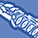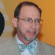cancel
Showing results for
Search instead for
Did you mean:New Contributor

## Enthalpy change stoichiometry

consider the synthesis reaction of C : 3A+2B>>> 4C

if the standard enthalpy change for this reaction is 500KJ/mol

what energy is needed to make 2.4moles of A react?

Tags (1)
2 RepliesContributor III

## Re: Enthalpy change stoichiometry

Dear Thierry,

If you know from the experiment and equation that the reaction requires 500KJ/mol A, then this reaction with only 2.4mol A would require 500KJ/mol A x 2.4mol A = 1200KJ. NOTE that you may be confusing the terms for change (per mole) and any absolute amound derived from a fixed amount of material. Be SURE that you have the problem correctly stated befoe proceeding with any solutions. NOte also that if you meant the enthalpy to be keyed to any other compound ('C' for example) the mathematical relationships would still apply (and the problem should be stated more clearly). Only the ratios will be different. That is, if it means 500KJ/mol of C produced, then you still take the ratio of A that forms C - 3:4. Now if you only have 2.4mol A, would would only produce (2.4/3) x 4 = 3.2 mol C. In these terms the heat required would then be 3.2 x 500 = 1600KJ. Careful defintion of the terms and conditions of an experiment or equation is necessary to arrive at a valid and useful solution.

Best regards,

StevenNew Contributor II

## Re: Enthalpy change stoichiometry

This seems like a simple question, on the surface. But it is a bit more subtle than it first appears. The subtle character revolves around the meaning of "500 kJ/mol." Per mole of what? Of "A" or "B" or "C"? People who do thermodynamic measurements have a somewhat unique perspective on how the term "mole" is used. As I have learned from working with them,this term is used in the sense of "mole of reaction." I.e., if three moles of "A" react with two moles of "B" to give four moles of "C", 500 kJ of energy in the form of heat would be released. Thus you get 500 kJ for three moles of "A." This means you get 2.4/3 times 500 kJ for 2.4 moles of "A."

Let me offer my opinion about the way the question was originally written. To the best of my knowledge, "A" has never reacted with "B." They sometimes combine to form words such as "abysmal." If our goal is to teach chemistry, we should use examples from the field. P4 reacting with O2, or, using an example from my experience, B5H9 reacting with O2 to give about 8800 kJ per mole of reaction. Or Mg reacting with N2, under appropriately "warm" conditions to form Mg3N2.